Question Pool Circles

# Circles - SAMAGRA Question Pool & Answers | Class 10 English Medium

Kerala Syllabus SAMAGRA SCERT SAMAGRA Question Pool for Class 10 English Medium Maths CirclesQn 1.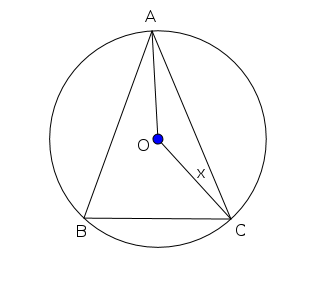A,B, C are points in the circle with centre O. If ∠OCA = x then

Find ∠OAC

Prove that ∠OCA + ∠ABC = 90°

Get Free Study Materials + 1 Week Free Trial of BrainsPrep Class 10 English Medium Tuition

Qn 2.

In the circle with centre O , ∠ CAD= 40° then

Find ∠B, and ∠ACD?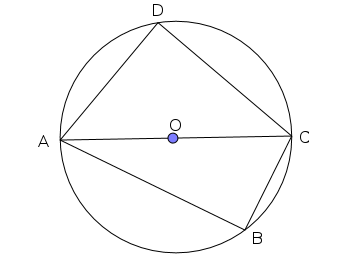∠B = ∠D= 90°

∠ACD =

Get Free Study Materials + 1 Week Free Trial of BrainsPrep Class 10 English Medium Tuition

Qn 3.

In the figure O is the centre of the circle. And ∠ADB=120° ,∠OAC=30° , Then

Find ∠ACB

Find ∠OAB

Justify that ABC is an equilateral Triangle.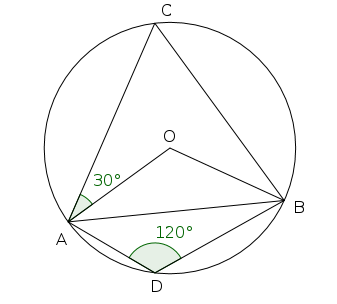∠C=180-120=60°                        &

Get Free Study Materials + 1 Week Free Trial of BrainsPrep Class 10 English Medium Tuition

Qn 4.

In the figure ∠C= 40°,∠OBC=15°

Find ∠AOB

Find ∠OAB

Find all angles of triangle ABC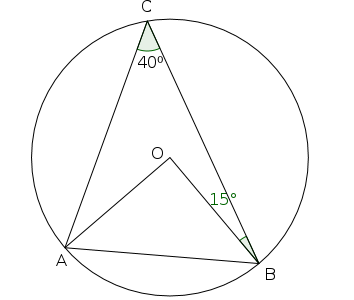Get Free Study Materials + 1 Week Free Trial of BrainsPrep Class 10 English Medium Tuition

Qn 5.

Draw a rectangle of length 6cm and breadth 4cm

Construct a square having same area of the rectangle.

Get Free Study Materials + 1 Week Free Trial of BrainsPrep Class 10 English Medium Tuition

Qn 6.

In the figure PA=PC, Which are the triangles formed when AC and BD are joined ?

Prove that ABDC is an isosceles trapezium?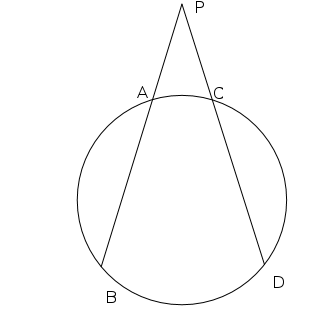a) ?PAC, ?PBD

Get Free Study Materials + 1 Week Free Trial of BrainsPrep Class 10 English Medium Tuition

Qn 7.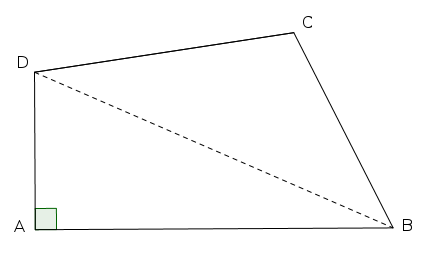• In the figure if we draw a circle with diagonal BD of the quadrilateral ABCD as diameter , where will be the positions of the vertices A and C  (∠ C =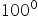)?

A is on the circle and  C is in the

Get Free Study Materials + 1 Week Free Trial of BrainsPrep Class 10 English Medium Tuition

Qn 8.

Draw a circle with radius 3 cm .Construct a triangle with vertices on the circle and having angles 50° , 60° , 70°

Get Free Study Materials + 1 Week Free Trial of BrainsPrep Class 10 English Medium Tuition

Qn 9.

In the figure the chords MA and NB extended and met  at P. MA=5cm , PA=7cm and PB=6cm.Calculate the length of NB?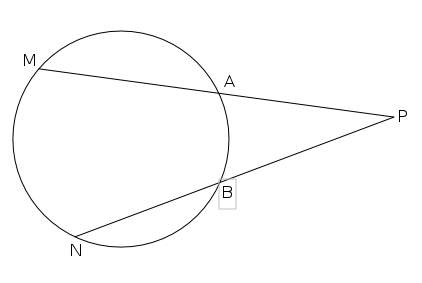MP=12 cm

PA xPM= PB xPN

PN=14cm

NB=8

Get Free Study Materials + 1 Week Free Trial of BrainsPrep Class 10 English Medium Tuition

Qn 10.

a) What is the measure of ∠ADC ?

b) Find the radius of the circle.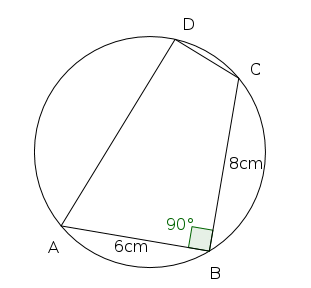b) diameter = 10 cm

Get Free Study Materials + 1 Week Free Trial of BrainsPrep Class 10 English Medium Tuition

Qn 11.

In the figure ?ABC is equilateral. BD=CD, AC=12cm and CD=5cm.Then

Find the measure of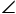ACB

Find the measure ofD

Find the measure ofBCD

Calculate the diameter of the circle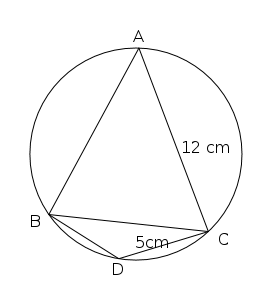a) ∠ACB =60°

b) ∠D =120°

Get Free Study Materials + 1 Week Free Trial of BrainsPrep Class 10 English Medium Tuition

Qn 12.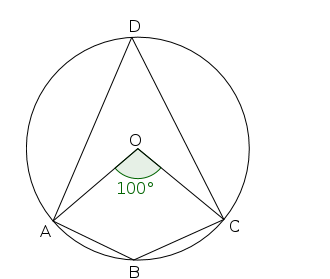In the figure O is the centre of the circle. If ∠AOC=100°

find ∠ABC ?

Get Free Study Materials + 1 Week Free Trial of BrainsPrep Class 10 English Medium Tuition

Qn 13.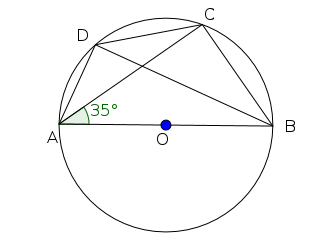In the figure ∠BAC = 35° find the measures of ∠BDC and ∠ADC?

∠BDC = 35°

Get Free Study Materials + 1 Week Free Trial of BrainsPrep Class 10 English Medium Tuition

Qn 14.

In the figure O is the centre of the circle. If ∠ AOB = 80° Find the measures of ∠ OCB and ∠ OBC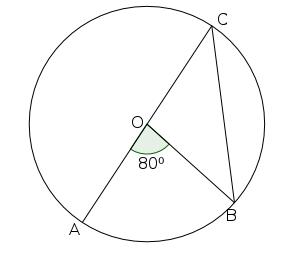Get Free Study Materials + 1 Week Free Trial of BrainsPrep Class 10 English Medium Tuition

Qn 15.
• In the figure of a clock , numbers 12 , 7 , and 5 are joined to form  a triangle.

(a) What are the measure of the angles of this triangle ?

(b) Give a suitable name for this triangle.

(c) Howmany such triangles can be drawn in this clock ?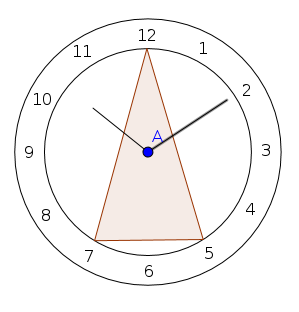• Angles are 75° , 75° , 30°
• Isosceles triangle
• 12

Get Free Study Materials + 1 Week Free Trial of BrainsPrep Class 10 English Medium Tuition

Qn 16.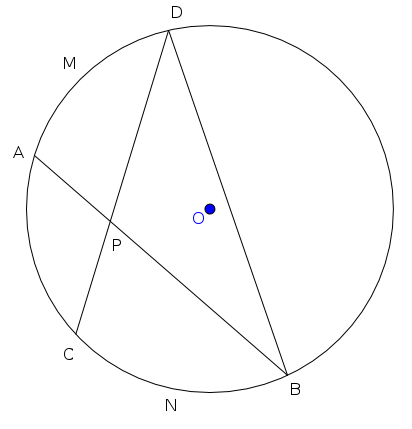• In the figure the length of the arc CNB is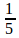of the perimetre of the circle and the length of the arc AMD is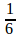of the perimetre of the circle.

(a) What is the measure of centre angle of the arc CNB ?

(b) Find the measure of ∠ CDB ?

(c) Find the measurement of ∠ ABD.

(d) Write the measurement of ∠ APD.

• Centre angle of arc CNB  = 72°
• ∠ CDB = 36°
• ∠ ABD =

Get Free Study Materials + 1 Week Free Trial of BrainsPrep Class 10 English Medium Tuition

Qn 17.
• In the figure chords CE , GD , CF are extended to meet outside the circle  at A and B. The lengths AG and BD are equal.

If AE x AC = AG x AD

(a) Write the product equal to BF x BC?

(b) Prove that AE x AC = BF x BC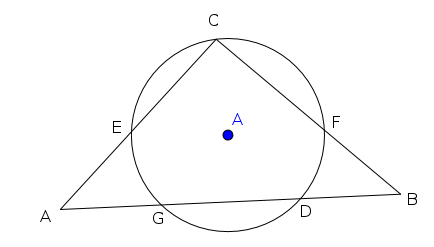• BD x BG
• AG x AD = BD x BG
• BF x BC = AG x AD
• BF x BC = AE x

Get Free Study Materials + 1 Week Free Trial of BrainsPrep Class 10 English Medium Tuition

Qn 18.
• In the figure O is the centre of the circle and ED is its diametre.

If ∠EGP = 67°

(a) What is the measure of ∠ EDP.

(b) Find other two angles of Δ ODP ?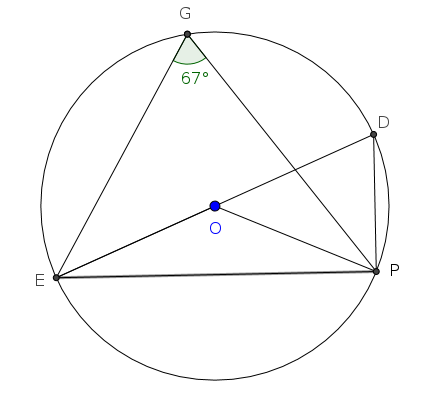a) ∠ EDP= 67°

b) ∠ DOP = 46° , ∠ OPD =

Get Free Study Materials + 1 Week Free Trial of BrainsPrep Class 10 English Medium Tuition

Qn 19.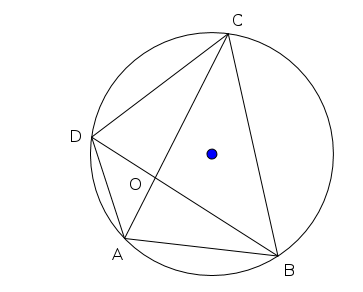Based on the figure find the angles from Part 2 which is equal to the angles in Part 1

 Part 1 Part 2 ∠ACB ∠BDC ∠ABD ∠AOD ∠BAC ∠ADB ∠ACD

∠ABD = ∠ACD

∠BAC =

Get Free Study Materials + 1 Week Free Trial of BrainsPrep Class 10 English Medium Tuition

Qn 20.

In the figure O is the centre of the circle and AB is the diametre. If ∠BOC = 120° , Find ∠ OCA and ∠ OAC ?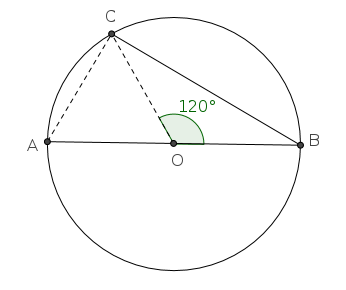∠ OCA = ∠ OAC = 60°

Get Free Study Materials + 1 Week Free Trial of BrainsPrep Class 10 English Medium Tuition

Qn 21.

In the figure O is the centre of the circle. ?ABC is  equilateral

Find the measures of

a) ∠A

b) ∠BOC

a) ∠A=

Get Free Study Materials + 1 Week Free Trial of BrainsPrep Class 10 English Medium Tuition

Qn 22.

In the figure PC=10 cm,CD=4cm, and PB:PA=2:3. Then

a) Find the length of PD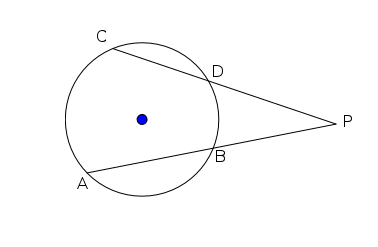b) Find the length of AB

Get Free Study Materials + 1 Week Free Trial of BrainsPrep Class 10 English Medium Tuition

Qn 23.

In the circle the chords AB and CD intersect at E. The central angle of arc BQC is 130°. The central angle of arc APD is 40° . Find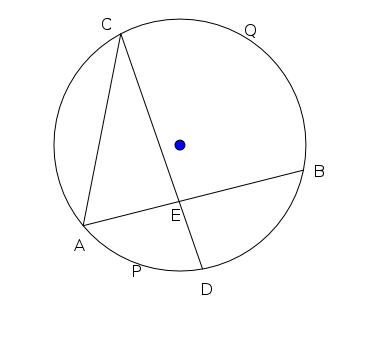a) ∠ACE

b) ∠CAE

c) ∠BEC

a) ∠ACE = 20°

b) ∠CAE = 65°

c) ∠BEC=

Get Free Study Materials + 1 Week Free Trial of BrainsPrep Class 10 English Medium Tuition

Qn 24.

Based on the figure write the angles from ? BPD equal to the following angles in ? APC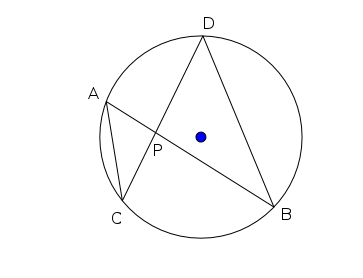a) ∠ACP

b) ∠CAP

a) ∠ACP = ∠PBD

b) ∠CAP = ∠

Get Free Study Materials + 1 Week Free Trial of BrainsPrep Class 10 English Medium Tuition

Qn 25.

In the figure PA=9cm, PB=4cm, and PC is 9cm more than PD

(a)  If PD = x find the length of PC ?(b)  Find the length of PD ?

(a) PD=x , PC= x+9

(b) PA x PB = PC x PD

9 x 4 = ( x + 9 )x

x² +

Get Free Study Materials + 1 Week Free Trial of BrainsPrep Class 10 English Medium Tuition

Qn 26.
• In the figure O is the centre of the circle and PQ is its diametre.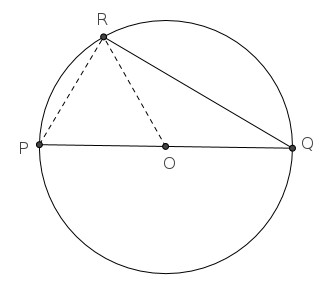If PR = OR

(a) Prove that Δ OPR is an equilateral triangle.

(b) Find all the angles of Δ OQR.

Get Free Study Materials + 1 Week Free Trial of BrainsPrep Class 10 English Medium Tuition

Qn 27.

In the figure ABCD is a quadrilateral .If a circle is drawn through A,B,and D state the position of the point C as Outside the circle,Inside the circle,or On the circle? Justify your answer.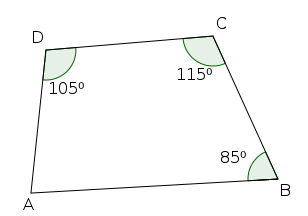∠A=55°

∠A + ∠C < 180

C is outside the

Get Free Study Materials + 1 Week Free Trial of BrainsPrep Class 10 English Medium Tuition

Qn 28.

In the figure ∠ AED=40° then

Which of the following can be  the measure of ∠ABC?

(140°, 130° , 150°, 180°)

Using the above measure of ∠ABC , find the measures of  angels of ?EAD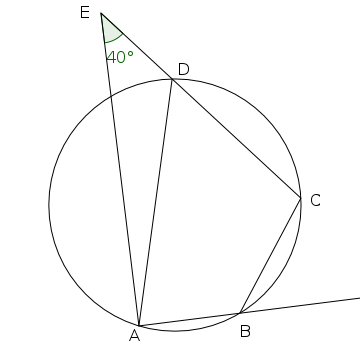∠ ABC=130° ( ∠ABC + ∠E < 180)

Get Free Study Materials + 1 Week Free Trial of BrainsPrep Class 10 English Medium Tuition

Qn 29.

In the figure AB is the diameter of the semicircle. IF AB = 9 cm, PB = 3 cm then

a) find PA ?

b) find PC² ?

c) Draw a square of area 18cm²?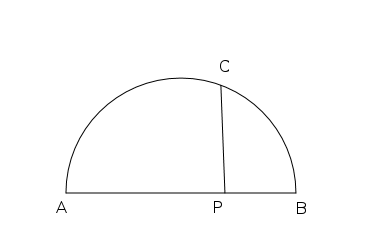a) PA = 6 cm

b) PC² = PA x PB = 6 x 3 = 18

Get Free Study Materials + 1 Week Free Trial of BrainsPrep Class 10 English Medium Tuition

Qn 30.

In the figure P,Q,R,S are points on a circle. Find all angles of quadrilateral PQRS?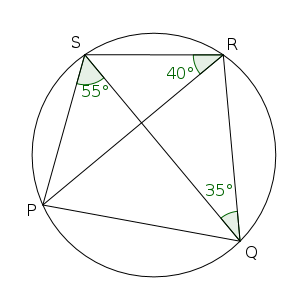∠PSR = 105°

∠ SPQ =85°

∠PQR=75°

∠QRS=95°

Get Free Study Materials + 1 Week Free Trial of BrainsPrep Class 10 English Medium Tuition

Qn 31.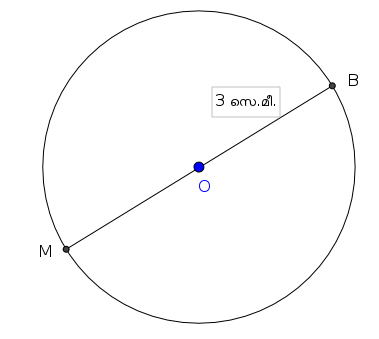•         Draw the  figure in your paper.

(a) Mark a point C on the circle with ∠ MBC = 30°

(b) Join M , B , C to get a triangle .

(c) Find other two angles of the triangle MBC

(d) Write the ratio of the smallest side to the radius of this triangle.

Get Free Study Materials + 1 Week Free Trial of BrainsPrep Class 10 English Medium Tuition

Qn 32.
• In the figure O is the centre and AB is the diametre of the circle. PC is perpendicular to AB.  If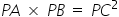• (a) What is the length of OP ?

(b) Find the length of PC .

(c) Write the ratio of the areas of Δ PBC and Δ APC ?

(d) Find the area of quadrilateral ACBD.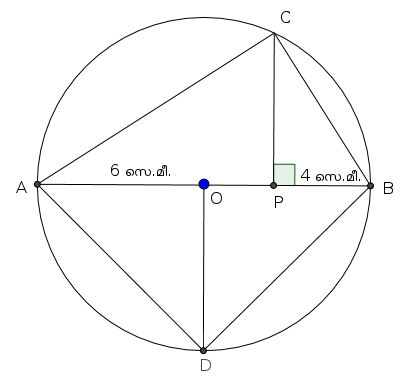(a) OP = 2cm.

(b) PC = √32

(c) For finding the ratio as 1 : 2

(d) 36 +

Get Free Study Materials + 1 Week Free Trial of BrainsPrep Class 10 English Medium Tuition

Qn 33.

A, B, and C are points on the circle with centre O . If ∠A = 60° , BC = 4cm then

Find ∠BOC

(2) When ∠A = 30°, Prove that BC is equal to circumradius.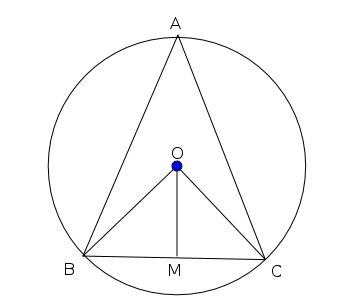Get Free Study Materials + 1 Week Free Trial of BrainsPrep Class 10 English Medium Tuition

Qn 34.

In the figure the diameter of the larger semi circle is 13 cm AP=8cm, PQ = 4 cm.

(a) Then PA x PB =.............

(b) PB = ....................

(c) Find the radius of the smaller semicircle?

(d) What is the area of the square BMRS?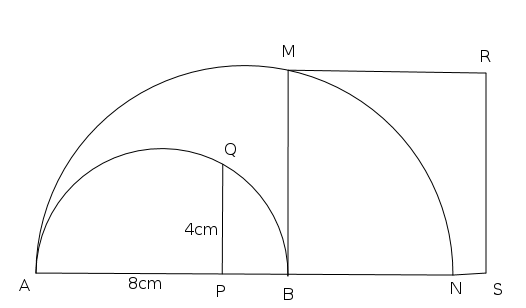(a) PA xPB=PQ²   = 16

(b) PB=2

(c) Radius of the small semicircle =5 cm

Get Free Study Materials + 1 Week Free Trial of BrainsPrep Class 10 English Medium Tuition